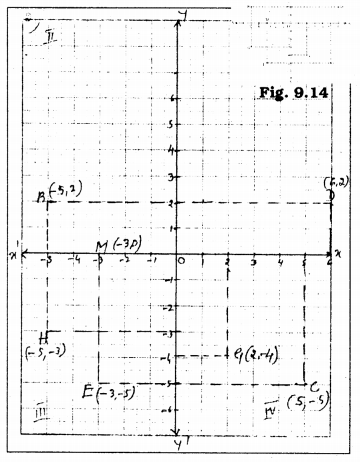# KSEEB Solutions for Class 9 Maths Chapter 9 Coordinate Geometry Ex 9.2

In this chapter, we provide KSEEB SSLC Class 9 Maths Chapter 9 Coordinate Geometry Ex 9.2 for English medium students, Which will very helpful for every student in their exams. Students can download the latest KSEEB SSLC Class 9 Maths Chapter 9 Coordinate Geometry Ex 9.2 pdf, free KSEEB SSLC Class 9 Maths Chapter 9 Coordinate Geometry Ex 9.2 pdf download. Now you will get step by step solution to each question.

## Karnataka Board Class 9 Maths Chapter 9 Coordinate Geometry Ex 9.2

Question 1.
Write the answer of each of the following questions :
(i) What is the name of horizontal and the vertical lines drawn to determine the position of any point in the Cartesian plane ?
(ii) What is the name of each part of the plane formed by these two lines?
(iii) Write the name of the point where these two lines intersect.
Solution:
(i) In a Cartesian plane, to determine the position of any point, the horizontal line is called the x-axis. A vertical line is y-
The y-coordinate is also called the ordinate.
Horizontal line → xOx’
Vertical line → yOy’

(ii) The name of each part of the plane formed by these two lines is called Quadrant.

(iii) The name of the point where these two lines intersect is called the origin. Coordinates of origin are (0. 0).

Question 2.
See Fig.9.14, and write the following:Solution:

1. The coordinates of B : (-5, +2)
2. The coordinates of C. : (+5, -5)
3. The point identified by the coordinates (-3, -5) : E.
4. The point identified by the coordinates (2, -4) : G.
5. The abscissa of the point D : 6
6. The ordinate of the point H: -3
7. The coordinates of the point L : (0, +5)
8. The coordinates of the point M : (-3, 0).

All Chapter KSEEB Solutions For Class 9 Maths

—————————————————————————–

All Subject KSEEB Solutions For Class 9

*************************************************

I think you got complete solutions for this chapter. If You have any queries regarding this chapter, please comment on the below section our subject teacher will answer you. We tried our best to give complete solutions so you got good marks in your exam.

If these solutions have helped you, you can also share kseebsolutionsfor.com to your friends.

Best of Luck!!